# 定向光struct Light
{
// vec3 position; // 现在不在需要光源位置了，因为它是无限远的
vec3 direction;
vec3 ambient;
vec3 diffuse;
vec3 specular;
};
...
void main()
{
vec3 lightDir = normalize(-light.direction);
...
}


for(GLuint i = 0; i < 10; i++)
{
model = glm::mat4();
model = glm::translate(model, cubePositions[i]);
GLfloat angle = 20.0f * i;
model = glm::rotate(model, angle, glm::vec3(1.0f, 0.3f, 0.5f));
glUniformMatrix4fv(modelLoc, 1, GL_FALSE, glm::value_ptr(model));
glDrawArrays(GL_TRIANGLES, 0, 36);
}


GLint lightDirPos = glGetUniformLocation(lightingShader.Program, "light.direction");
glUniform3f(lightDirPos, -0.2f, -1.0f, -0.3f);


Important

c++ if(lightVector.w == 0.0) // 请留意浮点数错误 // 执行定向光照计算

else if(lightVector.w == 1.0) // 像上一个教程一样执行顶点光照计算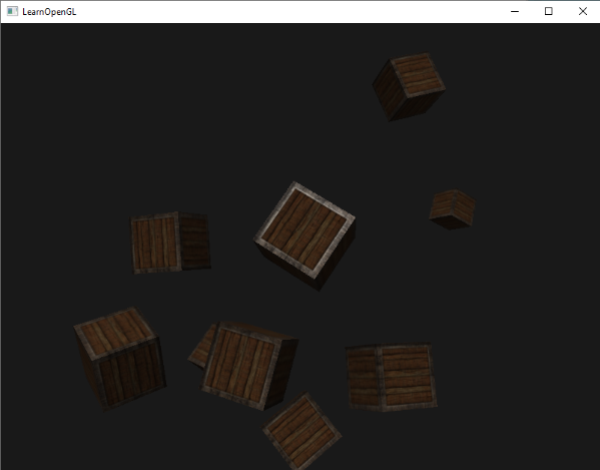# 点光源## 衰减

• 常数项通常是1.0，它的作用是保证分母永远不会比1小，因为它可以利用一定的距离增加亮度，这个结果不会影响到我们所寻找的。
• 一次项用于与距离值相乘，这会以线性的方式减少亮度。
• 二次项用于与距离的平方相乘，为光源设置一个亮度的二次递减。二次项在距离比较近的时候相比一次项会比一次项更小，但是当距离更远的时候比一次项更大。### 选择正确的值

7 1.0 0.7 1.8
13 1.0 0.35 0.44
20 1.0 0.22 0.20
32 1.0 0.14 0.07
50 1.0 0.09 0.032
65 1.0 0.07 0.017
100 1.0 0.045 0.0075
160 1.0 0.027 0.0028
200 1.0 0.022 0.0019
325 1.0 0.014 0.0007
600 1.0 0.007 0.0002
3250 1.0 0.0014 0.000007

### 实现衰减

struct Light
{
vec3 position;
vec3 ambient;
vec3 diffuse;
vec3 specular;
float constant;
float linear;
};


glUniform1f(glGetUniformLocation(lightingShader.Program, "light.constant"), 1.0f);


float distance = length(light.position - FragPos);
float attenuation = 1.0f / (light.constant + light.linear*distance +light.quadratic*(distance*distance));


Important

ambient *= attenuation;
diffuse *= attenuation;
specular *= attenuation;## 聚光

OpenGL中的聚光用世界空间位置，一个方向和一个指定了聚光半径的切光角来表示。我们计算的每个片段，如果片段在聚光的切光方向之间（就是在圆锥体内），我们就会把片段照亮。下面的图可以让你明白聚光是如何工作的：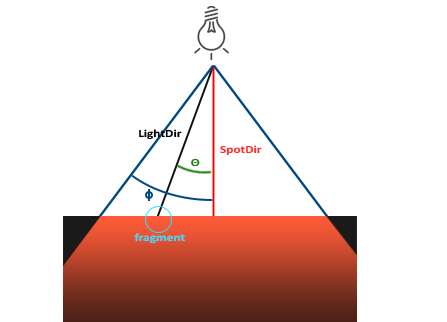• LightDir：从片段指向光源的向量。
• SpotDir：聚光所指向的方向。
• Phi$\phi$：定义聚光半径的切光角。每个落在这个角度之外的，聚光都不会照亮。
• Theta$\theta$LightDir向量和SpotDir向量之间的角度。$\theta$值应该比$\Phi$值小，这样才会在聚光内。

## 手电筒

struct Light
{
vec3 position;
vec3 direction;
float cutOff;
...
};


glUniform3f(lightPosLoc, camera.Position.x, camera.Position.y, camera.Position.z);
glUniform3f(lightSpotdirLoc, camera.Front.x, camera.Front.y, camera.Front.z);


float theta = dot(lightDir, normalize(-light.direction));
if(theta > light.cutOff)
{
// 执行光照计算
}
else // 否则使用环境光，使得场景不至于完全黑暗
color = vec4(light.ambient*vec3(texture(material.diffuse,TexCoords)), 1.0f);


Important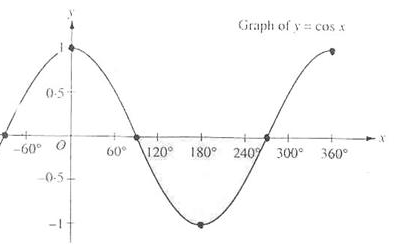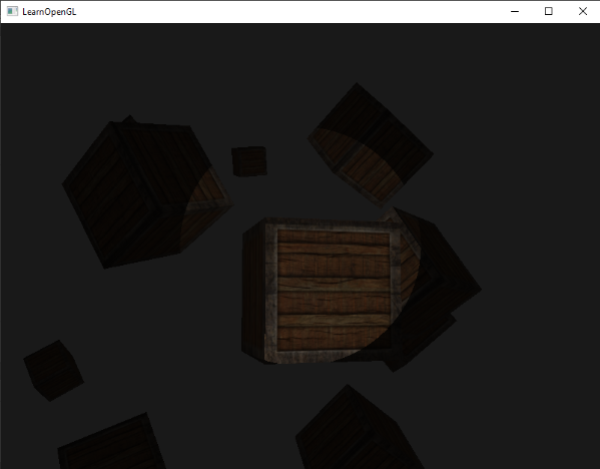## 平滑/软化边缘

$\theta$ $\theta$（角度） $\phi$（内切） $\phi$（角度） $\gamma$（外切） $\gamma$（角度） $\epsilon$ $I$
0.87 30 0.91 25 0.82 35 0.91 - 0.82 = 0.09 0.87 - 0.82 / 0.09 = 0.56
0.9 26 0.91 25 0.82 35 0.91 - 0.82 = 0.09 0.9 - 0.82 / 0.09 = 0.89
0.97 14 0.91 25 0.82 35 0.91 - 0.82 = 0.09 0.97 - 0.82 / 0.09 = 1.67
0.97 14 0.91 25 0.82 35 0.91 - 0.82 = 0.09 0.97 - 0.82 / 0.09 = 1.67
0.83 34 0.91 25 0.82 35 0.91 - 0.82 = 0.09 0.83 - 0.82 / 0.09 = 0.11
0.64 50 0.91 25 0.82 35 0.91 - 0.82 = 0.09 0.64 - 0.82 / 0.09 = -2.0
0.966 15 0.9978 12.5 0.953 17.5 0.966 - 0.953 = 0.0448 0.966 - 0.953 / 0.0448 = 0.29

float theta = dot(lightDir, normalize(-light.direction));
float epsilon = light.cutOff - light.outerCutOff;
float intensity = clamp((theta - light.outerCutOff) / epsilon,0.0, 1.0);
...
// We’ll leave ambient unaffected so we always have a little
light.diffuse* = intensity;
specular* = intensity;
...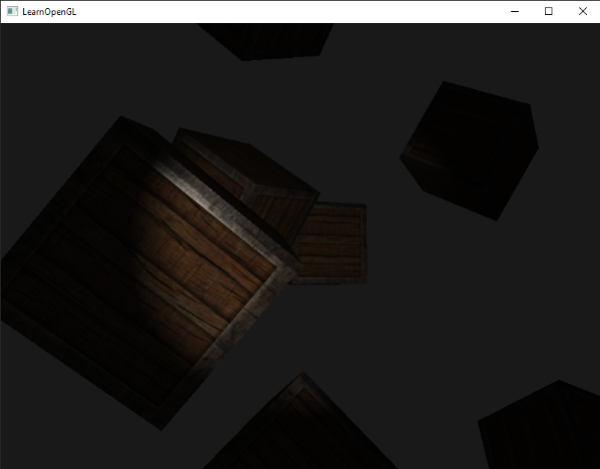## 练习

• 试着修改上面的各种不同种类的光源及其片段着色器。试着将部分矢量进行反向并尝试使用 < 来代替 > 。试着解释这些修改导致不同显示效果的原因。